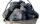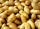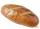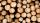# Excavator

The excavator bucket capacity is 0.5 m3. Determine the mass of sand that the excavator picks up. The sand density is 1650 kg/m3.

Result

m =  825 kg

#### Solution:Leave us a comment of example and its solution (i.e. if it is still somewhat unclear...):

Showing 0 comments:Be the first to comment!#### To solve this example are needed these knowledge from mathematics:

Tip: Our volume units converter will help you with converion of volume units.

## Next similar examples:

1. Tons of coalCoal hopper has a capacity of 285 liters. How many tons is it? The bulk density of coal is 916 kg/m3.
2. Liter of goldWhat weight does 1 dm3 of gold have? Gold density is 19,300 kg / m3
3. Solid in waterThe solid weighs in air 11.8 g and in water 10 g. Calculate the density of the solid.
4. PotatoesCould 446 tons of potatoes (ρ = 771 kg/m³) fits in a warehouse with a volume of 699 m³ ?
5. RecipeA recipe requires 2 pounds of flour. If a chef wants to triple the recipe, how many ounces of flour will be needed?
6. Pound2kilosHow many pounds make 1 kilograms?
7. Bread5 of the same bread has the same weight as three bread and 4 kg of fruit. What weight has one bread?
8. WoodWood density is 0.6 g/cm3. How many kilograms weight 1 m3 of wood?
9. BeerWhich beer is better to drink: small beer (0.3 L) for 0.67 € or large (0.5 L) for 1.81 €?
10. Water 31Richard takes 3 1/6 liters of water before noon and 2 3/5 liters of water after noon. How many litres of water does Richard consume a day ?
11. Passenger boatTwo-fifths of the passengers in the passenger boat were boys. 1/3 of them were girls and the rest were adult. If there were 60 passengers in the boat, how many more boys than adult were there?
12. Find xSolve: if 2(x-1)=14, then x= (solve an equation with one unknown)
13. Simple equationSolve the following simple equation: 2. (4x + 3) = 2-5. (1-x)
14. Negative in equation2x + 3 + 7x = – 24, what is the value of x?
15. The shopThe shop has 3 hectoliters of water. How many liter bottles is it?
16. Pizza 4Marcus ate half pizza on monday night. He than ate one third of the remaining pizza on Tuesday. Which of the following expressions show how much pizza marcus ate in total?
17. Cupcakes 2Susi has 25 cupcakes. She gives 4/5. How much does she have left?